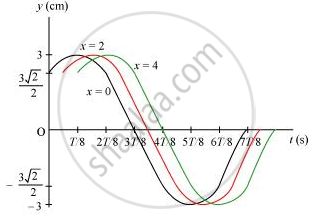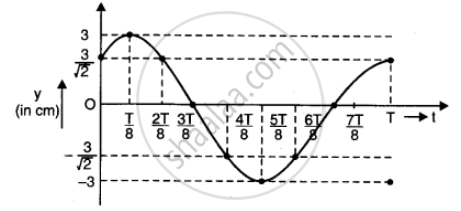# For the Wave Described in Exercise 15.8, Plot the Displacement (Y) Versus (T) Graphs for X = 0, 2 and 4 Cm. What Are the Shapes of These Graphs? in Which Aspects Does the Oscillatory Motion in Travelling Wave Differ from One Point to Another: Amplitude, Frequency Or Phase? - Physics

For the wave described in Exercise 15.8, plot the displacement (y) versus (t) graphs for x = 0, 2 and 4 cm. What are the shapes of these graphs? In which aspects does the oscillatory motion in travelling wave differ from one point to another: amplitude, frequency or phase?

#### Solution 1

All the waves have different phases.

The given transverse harmonic wave is:

y (x,t)= 3.0 sin (36t + 0.018x + pi/4) ...(i)

For x = 0, the equation reduces to:

y (0,t) = 3.0 sin (36t + pi/4)

Also, omega = (2pi)/T = 36 " rad/s"^(-1)

:. T = pi/8 s

Now, plotting y vs. t graphs using the different values of t, as listed in the given table.

 t(s) 0 T/8 2T/8 3T/8 4T/8 5T/8 6T/8 7T/8 y(cm) (3sqrt2)/2 3 (3sqrt2)/2 0 (-3sqrt2)/2 -3 (-3sqrt2)/2 0

For x = 0, x = 2, and x = 4, the phases of the three waves will get changed. This is because amplitude and frequency are invariant for any change in x. The y-t plots of the three waves are shown in the given figure.#### Solution 2

The transverse harmonic wave is

y(x,t) = 3.0 sin (36t + 0.018x + pi/4)

for x = 0

y(0,t) = 3 sin(36t + 0 + pi/4) = 3 sin (36t + pi/4)  ...1

Here omega = (2pi)/T = 36 => T =(2pi)/36

To plot a(y) versus (t) graph, different values of y corresponding to the values of t may be tabulated as under (by making use of equation 1)

 t(s) 0 T/8 2T/8 3T/8 4T/8 5T/8 6T/8 7T/8 T y(cm) (3sqrt2)/2 3 (3sqrt2)/2 0 (-3sqrt2)/2 -3 (-3sqrt2)/2 0 3/sqrt2

Using the values of t and y (as in the table), a graph is plotted as under The graph obtained is sinusoidal.
Similar graphs are obtained for y x = 2 cm and x = 4 cm. The (incm) oscillatory motion in the travelling wave only differs in respect of phase. Amplitude and frequency of oscillatory motion remains the same in all the cases.Concept: The Speed of a Travelling Wave
Is there an error in this question or solution?

#### APPEARS IN

NCERT Class 11 Physics
Chapter 15 Waves
Q 9 | Page 387